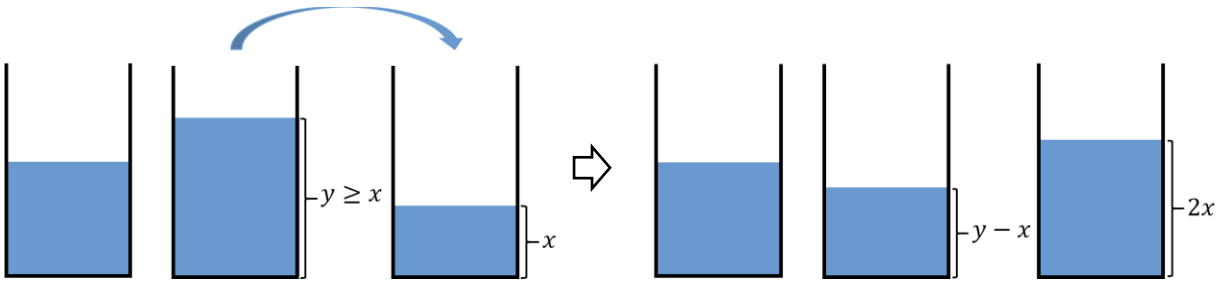시간 제한메모리 제한제출정답맞힌 사람정답 비율
1 초 (추가 시간 없음) 1024 MB59311850.000%

## 문제You are one of the $456$ players participating in a series of children’s games with deadly penalties. Passing through a number of maze-like hallways and stairways, you have opened the gate of the next game. There are three buckets with infinite capacity, each of which contains an integral number of liters of water. The buckets are numbered from $1$ to $3$. The amounts of water initially contained in buckets $1$, $2$, and $3$ are given as $X$, $Y$, and $Z$, respectively.

At any time, you can double the amount of one bucket by pouring into it from another one. Specifically, you can pour from a bucket of $y$ liters into one of $x$ ($≤ y$) liters until the latter contains $2x$ liters and the former does $y - x$ liters. Note that $x$ and $y$ are always integers and $x ≤ y$. See the Figure J.1.Figure J.1 A process of pouring

In order to survive, you have to empty one of the buckets in a limited number of pouring. Fortunately, it is always possible to empty one of the buckets. Given the initial amounts $X$, $Y$, and $Z$ of water in three buckets, write a program to output a sequence of pouring until one of the buckets is empty for the first time.

## 입력

Your program is to read from standard input. The input starts with a line containing three integers $X$, $Y$, and $Z$ ($1 ≤ X ≤ Y ≤ Z ≤ 10^9$), representing the initial amounts of water in buckets $1$, $2$, and $3$, respectively.

## 출력

Your program is to write to standard output. The first line should contain the number $m$ of pouring until one of the buckets is empty for the first time. The number $m$ should be no more than $1,000$. Each of the following $m$ lines contains two integers $A$ and $B$ ($1 ≤ A ≠ B ≤ 3$), which means you pour from bucket $A$ into bucket $B$ in a process of pouring. You should guarantee that one of buckets is empty for the first time after the $m$ pouring. If there are several ways to empty one of the buckets, then print one of them.

## 예제 입력 1

1 2 3


## 예제 출력 1

2
3 2
3 1


## 예제 입력 2

1 4 6


## 예제 출력 2

3
2 1
3 1
1 3